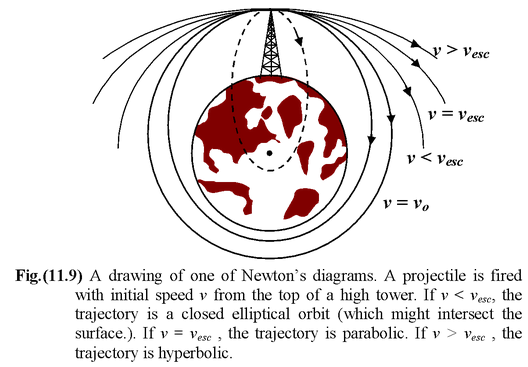# Escape Speed

## Escape Speed

1. It is the minimum velocity required to escape from the gravitational field of the planet.
2. When a rocket is fired with the minimum escape speed vesc it will reach r = ∞ with zero speed; that is, Ef = K + U = 0. Its initial energy at the earth's surface is
3. Ei =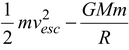4. Using energy conservation
5. Ef = Ei
6. O =7. or vesc = √2GM/R         (11.17)
8. Note that the escape speed does not depend on the mass of the rocket.
9. SubstitutingM = 6 × 1024 kg ; G = 6.67 × 10-11 Nm2/kg2
10. R = 6.4 × 106 m
11. we getvesc = 11.2 km/s
12. Example 11.4
13. A rocket is fired vertically with half the escape speed. What is its maximum altitude in terms of the radius of the earth R? Ignore the earth’s rotation.

### Solution

1. This problem can be solved easily by using the law of conservation of energy.
2. Note that one must take care not to use Ug = mgh since this equation is valid only when g may be taken to be a constant. The initial energy is
3. Ei =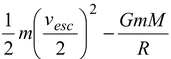4. =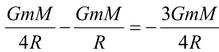5. At the maximum altitude, the kinetic energy is zero.
6. Ef = 0 -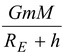7. On simplification, Ef = Ei, gives h = R/3

### Bound and Unbound Trajectories

1. Suppose that a cannonball is fired from the peak of very tall tower with speed v. Let us consider the shapes of the paths for various values of v.
2. If the ball is located at a distance r from the center of the earth, the speed that is required to escape from the point is given by
3. vesc = √2GM/r
4. For a circular orbit of radius r the orbital speed is vo = √GM/r
1. When v < vo, the orbit is elliptical, with the peak as the apogee (the farthest point from earth). If v is too small, the projectile will strike the earth.
1. When v = vo, the orbit is circular.
2. When vesc > v > vo, the orbit is again an ellipse, but now the peak is the perigee (the closest point to earth).
3. When v = vesc = √2 vo, the path is parabolic and is not a closed orbit. The object is not bound.
2. When v > vesc, the path is hyperbola and not closed. The object is not bound.
3.